# 3D Surface Rendering in Postscript

J. Scott Drader, Math 308, Fall 2003

# Contents

1. Introduction
2. Bezier Curve Review
1. Tensor Product Patches
2. Bezier Triangle Patches
3. Other Representations
4. Bibliography
5. surface3d.ps Reference

# 1. Introduction

Bezier curves have proven to be a very useful method of modeling surfaces in many applications. We will assume the reader is familiar with Bezier curves, Bernstein polynomials, and the DeCasteljau algorithm. We only review the definition and properties of Bezier curves, rather than derive them (section 1). We will focus on two surface representations based on Bezier curves (section 2); tensor product surfaces (section 3), and Bezier triangles (section 4). We will discuss the properties they inherit from Bezier curves, as well as methods for subdividing and rendering them. Finally we mention some other more complex representations not dealt with here and why they are desirable (section 5).

The final section details the provided libraries that were used to produce the images shown. The libraries provide methods for Bezier curves in 2D and 3D, as well for tensor product surfaces and Bezier triangles. The 3d portion the library is built on top of ps3d.inc.

# 2. Bezier Curve Review

#### Definition

Bezier curves can be described on a high-level by their geometric interpretation, and indeed this is the property that makes them so elegant. A Bezier curve is a function defined over a single parameter that interpolates a sequence of points. As the parameter changes, the path of the line created goes from the first point in the sequence to the last, moving a long curve that is influenced intuitively by the intermediate control points.

Recall that Bezier curves use the Bernstein polynomials as basis functions. The number of points that are interpolated determine the degree of the curve (and the degree of the polynomial that interpolates them). The equation of the curve is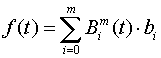where bi is the ith control point (i.e. the vector b is the control polygon) and Bim(t) is the ith Bernstein polynomial of degree m. As the parameter t varies from 0 to 1, f(t) traverses the curve.

#### Properties

Bezier curves have several key properties (inherent from the Bernstein polynomials) that are important to the properties curves and 3D surfaces based on them. As we are concerned with 3D surfaces we only state the properties and do not prove them.

1. The curve interpolates its first and last control point.

2. The entire curve lies within the convex hull of the control points.

3. It is invariant under affine transformations (i.e. transforming the curve is only a matter of transforming the control points)

4. The curve is tangent to its control polygon at the endpoints.

5. The curve can be evaluated and/or subdivided recursively by the DeCasteljau algorithm.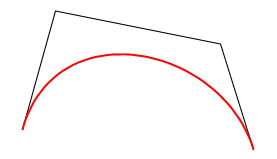A degree 3 (cubic) Bezier curve and its control

polygon.

#### Subdivision and Rendering

There are several methods of drawing Bezier curves. The most obvious method is to directly evaluate the polynomial at fixed intervals of t to produce a set of points on the curve, which can be joined to form an approximation of the curve. Although this method works, it is inefficient. The above method can be sped up by using the recursive DeCasteljau algorithm to evaluate the curve for values of t. The best method however is recursively subdividing the curve by repeated applications of DeCasteljau.

The DeCasteljau has the property that evaluation of the curve for a value of t produces control points for 2 smaller curves, one from 0 to t and the 2nd from t to 1. Hence applying it at t = 1/2 splits the curve into a left and right curve. By recursively splitting the original curve, the polygon formed by assembling the control points of the sub-curves quickly converges to the final curve.After 2 subdivisions, the curve is already closely approximated

by the control polygons of the 4 resultant curves.

# 3. Tensor Product Patches

#### Definition

Just as we can use a parameterization in one variable to define a curve, we can use a parameterization in 2 variables to define a surface in 3D. Tensor product surfaces (or rectangular Bezier patches) are the 2-variable extension of Bezier curves. Instead of interpolating a sequence of points, they can be thought as a way to interpolate a sequence of Bezier curves, or a rectangular mesh of control points. The formula iswhere bi,j is the i,jth control point in the control mesh (i.e. b is the 2D mesh of control points, which we will refer to as the control net). Holding the parameter s constant and traversing the surface for 0 < t < 1 traces a Bezier curve that lies on the surface, and vice versa.

#### Properties

The properties of tensor product surfaces are natural extensions of those for Bezier curves. They are listed below, and numbered such that they correspond to the above list of properties of Bezier curves.

1. The patch interpolates the corner vertices of its control net.

2. The surface lies within the convex hull of its control points.

3. It is invariant under affine transformations (i.e. transforming the surface is only a matter of transforming the control net).

4. At its corners, the surface is tangent to the vectors formed by the corner control point and the nearest control points on the adjacent edges.

5. The patch can be evaluated and/or subdivided recursively by applying the DeCasteljau algorithm once in each parameter direction.

6.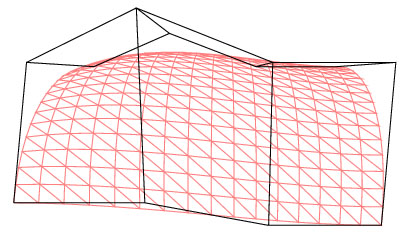A rectangular patch (degree 2 in s and 3 in t) and its control polygon.

#### Subdivision and Rendering

Rendering of tensor product surfaces is also analogous to the curve case, with the exception that everything has to be done twice, once in each parameter. We again have direct computation of the polynomial and direct computation with DeCasteljau's algorithm as options, but subdivision is attractive again. Just as a Bezier curve can be split into 2 separate but equivalent curves by a single application of the DeCasteljau algorithm, a rectangular patch surface can be split into 4 smaller but equivalent patches (a split in each parameter direction). The parameter which is chosen first does not affect the result. Rendering via subdivision is also fairly similar to the Bezier curve case. The obvious difference is that it is no longer a matter of simply connecting control points, and normal vectors are required for realistic shading.

There is more than one way to obtain a triangulation of the surface after having subdivided a patch into several sub-patches. One way is to render each sub-patch by creating a triangulation of its control mesh, and computing normals based on the vertices of the each resulting triangle. This method is fine when only a wireframe approximation of the surface is needed, or when normals only need to be provided

per-triangle rather than per-vertex.

A second and arguably more "correct" way is to render each sub-patch as two triangles (composed of the 4 corner control points of the subpatch). Note that this scheme does not make use of the interior control points patches, and hence would typically require an extra subdivision or two to obtain an equivalently dense triangle mesh. The reason it is often the favorable method is because exact normal vectors can be obtained at all vertices used in the triangulation (see property 4 above) which is ideal for renderers requiring per-vertex normals.The triangulation and normals after 2 subdivisions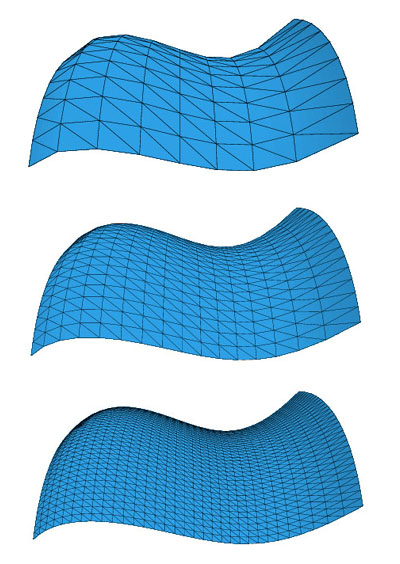Refinement of a rectangular patch through subdivision

# 4. Bezier Triangle Patches

#### Definition

Bezier triangles are not as simple as tensor product patches. Rather than using s and t as parameters as in the tensor patch case, points on the curve are parameterized with Barycentric coordinates. Given a parameter domain bounded by v1, v2, and v3, a point x inside the domain can be described as a weighting of the vi :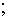Given this parameterization, the basis functions required are the 3-dimensional Bernstein polynomials, which are defined as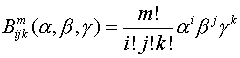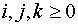and this gives us the final equation for the curve: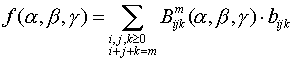#### Properties

The properties we are interested in are virtually identical to those listed above for tensor product surfaces, with the notable difference that the DeCasteljau algorithm has a slightly different form with the Barycentric parameterization.

#### Subdivision and Rendering

The DeCasteljau algorithm in this case subdivides the control mesh into 3 sub-meshes as follows: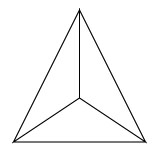Unlike with Bezier curves or tensor product patches however, repeated applications of this subdivision scheme do not produce a triangulation that approaches the original surface since the edges of the patch are not refined. A more complex algorithm that splits the mesh into the configuration below is possible.

The naive algorithm to do so requires 12 calls to the DeCasteljau algorithm, but it can be done in 4 calls by using a clever sequence of splits.The triangulation and normals of a cubic Bezier triangle after 2 subdivisions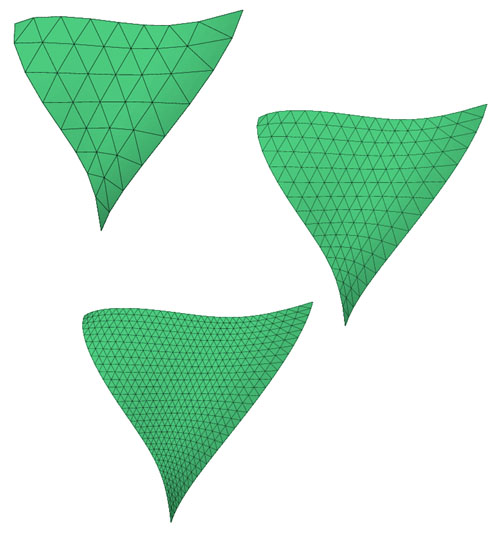Refinement of a triangular patch through subdivision

# 5. Other Representations

An often desirable but difficult to deal with task that we have not discussed involves joining curves or patches into more complex curves or patches. Typical reasons for combining several curves or patches include the desire to assemble more complex surfaces, and the desire to achieve more locality of control (i.e. a control point affects only the part of the curve closest to it). The fundamental problem that arises in attempting to to achieve either of these ideals is linking the curves or patches in a continuous fashion.

Constraints placed on neighboring curves or patches of the type we have discussed can be fairly easily derived for each of the the representations via their polar forms, but this is beyond our scope, and provides a good starting point for further reading. Representations that we have not mentioned that begin to deal with these problems by implicitly enforcing the continuity constraints include B-Splines, rational curves, NUBS (Non-Uniform B-Splines) and NURBS (Non-Uniform Rational B-Splines).

# 6. Bibliography

Gallier, J., Curves and Surfaces in Geometric Modelling, Morgan Kaufmann Publishers, Sanfrancisco, CA, 2000.

Akenine-Möller, T, Haines, E, Bezier Triangles and N-Patches, http://www.gamasutra.com/features/20020715/mollerhaines_01.htm

Kruger, G, Curved Surfaces Using Bezier Patches, http://www.gamasutra.com/features/19990611/bezier_01.htm

# 7. surface3d.ps Reference

surface3d.inc is a library for rendering Bezier curves, tensor product patches, and Bezier triangles in PostScript. Methods are provided for evaluation, subdivision, and rendering. surface3d.inc makes use of ps3d.inc and it is assumed they are in the same directory.

#### Structures

There are 4 main structures used by surface3d.inc. They are detailed below:

 Structure Name: bez3 Description: The control polygon for a 3d Bezier curve Structure: [ b1 ... bn ]; where the degree of the curve is n -1

 Structure Name: rpatch Description: The control polygon for a tensor product surface Structure: [[b1,1 ... b1,m] ... [bn,1 ... bn,m]]; where the patch is degree n -1 in s and m - 1 in t

 Structure Name: tpatch Description: The control polygon for a Bezier triangle Structure: [[b1,1] ... [bn,1 ... bn,n]]; where the patch is degree n -1

 Structure Name: frag Description: A triangular surface fragment. Structure: [[v1  n1]  [v2  n2]  [v3  n3]]; where vi are the vertices and ni their normal vectors

#### Methods

bez3 methods:

 Method Name: bez3-eval Description: Evaluates a Bezier curve at a given value of t Parameter(s): bez3 t; the Bezier and the parameter value Return Value(s): v; the point

 Method Name: bez3-subdiv Description: Subdivides a Bezier curve at a given value of t. Parameter(s): bez3 t; the Bezier and the parameter value Return Value(s): bez-left bez-right; the 2 resultant Beziers

 Method Name: bez3-subdiv-arr Description: Recursively subdivides an array of Beziers at t = 0.5 Parameter(s): bez3-arr n; the array of Beziers and the number of subdivisions to perform Return Value(s): bez3-arr-new; the new array of Beziers

 Method Name: bez3-build-path-arr Description: Builds a path for an array of Beziers Parameter(s): bez3-arr; the array of Beziers Return Value(s): none

rpatch methods:

 Method Name: rpatch-eval Description: Evaluates a rectangular patch for given values of s and t. Parameter(s): rpatch s t; the Bezier and the parameter values Return Value(s): v; the point

 Method Name: rpatch-subdiv Description: Subdivides a rectangular patch into 4 patches Parameter(s): rpatch; the patch Return Value(s): rpatch1 rpatch2 rpatch3 rpatch4; the 4 resultant patches

 Method Name: rpatch-subdiv-arr Description: Recursively subdivides an array of rectangular patches (into 4 patches each) Parameter(s): rpatch-arr n; the array of patches and the number of subdivisions to perform Return Value(s): rpatch-arr-new; the new array of patches after n subdivisions

 Method Name: rpatch-build-path-arr Description: Built a path representing the control meshes of an array of patches Parameter(s): rpatch-arr; the array of patches Return Value(s): none

 Method Name: rpatch-get-fragments-arr Description: Returns an array of fragments representing the array of patches Parameter(s): rpatch-arr; the array of patches Return Value(s): frag-arr; the array of fragments

tpatch methods:

 Method Name: tpatch-rotate Description: Rotates a patch rst to str Parameter(s): rst; the patch Return Value(s): str; the rotated patch

 Method Name: tpatch-transpose Description: Takes the patch rst and computes its transpose, rts Parameter(s): rst; the patch Return Value(s): rts; the transposed patch

 Method Name: tpatch-eval Description: Evaluates a patch for given Barycentric coordinates Parameter(s): tpatch [alpha beta gamma]; the patch and Barycentric coordinates Return Value(s): v; the point

 Method Name: tpatch-subdiv-3 Description: Subdivides a triangular patch into 3 patches for the given Barycentric coordinate Parameter(s): rst [alpha beta gamma]; the patch and Barycentric coordinates Return Value(s): ars ast atr; the 3 resultant patches

 Method Name: tpatch-subdiv-4 Description: Subdivides a patch into 4 patches for Barycentric coordinates [1/3 1/3 1/3] Parameter(s): rst; the patch to be subdivided Return Value(s): bas cra cab cbt; the 4 resultant patches

 Method Name: tpatch-subdiv-arr Description: Recursively subdivides an array of patches (into 4 patches each) Parameter(s): tpatch-arr n; the array of patches and the number of subdivisions to be performed Return Value(s): tpatch-arr-new; the new array of patches after n subdivisions

 Method Name: tpatch-get-fragments-arr Description: Returns an array of fragments representing the array of patches Parameter(s): tpatch-arr; the array of patches Return Value(s): frag-arr; the array of fragments

frag methods:

 Method Name: draw-fragments-gouraud Description: Draws an array of fragments with Gouraud shading Parameter(s): frag-arr light-source shade-func color; the array of fragments, a 4-value array reprsenting the light source, a 4-value array representing the shading curve, a 3-value array representing an RGB color Return Value(s): none

 Method Name: build-fragments-wireframe Description: Built a path representing the edges of an array of fragments Parameter(s): frag-arr; the array of fragments Return Value(s): none

 Method Name: build-fragment-normals Description: Built a path representing the normal vectors of an array of fragments Parameter(s): frag-arr len; the array of fragments, the length the normals are to be drawn Return Value(s): none